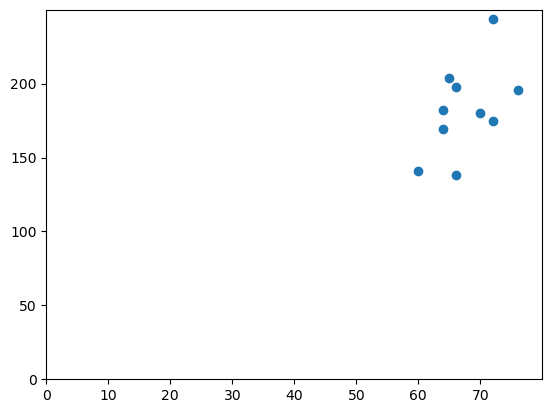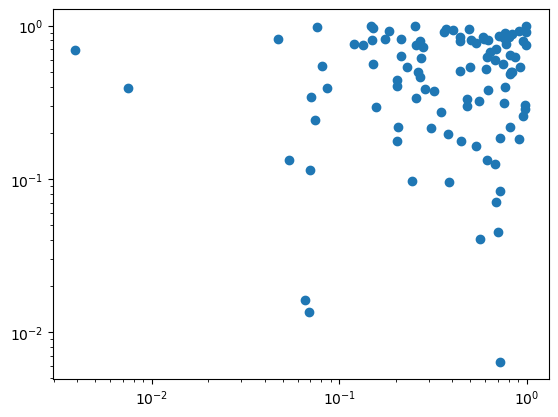# How to change axes, ticks, and scale in a plot (in Python, using Matplotlib)

See all solutions.

The mathematical markings and measurements in a plot can make a big difference on its readability and usefulness. These include the range of each axis, which points on that axis are marked with tick marks, and whether the axes use linear or logarithmic scaling. How can we customize these options?

Related topics:

## Solution

We will create some fake data using Python lists, for simplicity. But everything we show below works also if your data is in columns of a DataFrame, such as df['age'].

1
2
3
patient_id     = [   0,   1,   2,   3,   4,   5,   6,   7,   8,   9 ]
patient_height = [  60,  64,  64,  65,  66,  66,  70,  72,  72,  76 ]
patient_weight = [ 141, 182, 169, 204, 138, 198, 180, 175, 244, 196 ]


The conventional way to import matplotlib in Python is as follows.

1
import matplotlib.pyplot as plt


You can change the plot range and where tick marks are shown, in either the $x$ or $y$ directions (or both) as follows.

1
2
3
4
5
6
plt.scatter( patient_height, patient_weight )
plt.xlim( [ 0, 80 ] )          # Plot from x=0 to x=80.
plt.ylim( [ 0, 250 ] )         # Plot from y=0 to y=250.
plt.xticks( range(0,80,10) )   # Put x axis ticks every 10 units.
plt.yticks( range(0,250,50) )  # Y ticks every 50.  You can provide any list.
plt.show()If you need either axis to be logarithmically scaled, it takes just one line of code.

1
2
3
4
5
import numpy as np   # Just using this to make random data.
plt.scatter( np.random.rand(100), np.random.rand(100) )
plt.xscale( 'log' )  # You can include one of these two
plt.yscale( 'log' )  # lines, or both, or neither.
plt.show()